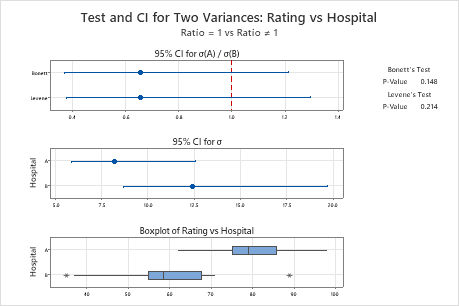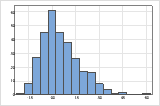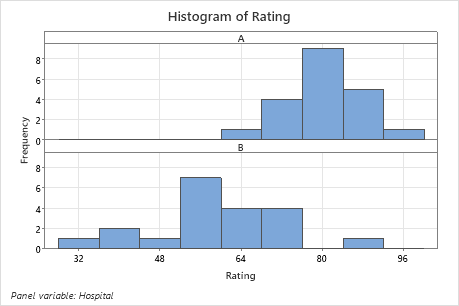# Interpret the key results for 2 Variances

Complete the following steps to interpret a 2 variances test. Key output includes the ratio, the confidence interval, the p-value, and several graphs.

## Step 1: Determine a confidence interval for the ratio of standard deviations or variances

First, consider the ratio in the sample variances or the sample standard deviations, and then examine the confidence interval.

The estimated ratio of standard deviations and variances of your sample data is an estimate of the ratio in population standard deviations and variances. Because the estimated ratio is based on sample data and not on the entire population, it is unlikely that the sample ratio equals the population ratio. To better estimate the ratio, use the confidence interval.

The confidence interval provides a range of likely values for the ratio between two population variances or standard deviations. For example, a 95% confidence level indicates that if you take 100 random samples from the population, you could expect approximately 95 of the samples to produce intervals that contain the population ratio. The confidence interval helps you assess the practical significance of your results. Use your specialized knowledge to determine whether the confidence interval includes values that have practical significance for your situation. If the interval is too wide to be useful, consider increasing your sample size. For more information, go to Ways to get a more precise confidence interval.

By default, the 2 variances test displays the results for Levene's method and Bonett's method. Bonett's method is usually more reliable than Levene's method. However, for extremely skewed and heavy tailed distributions, Levene's method is usually more reliable than Bonett's method. Use the F-test only if you are certain that the data follow a normal distribution. Any small deviation from normality can greatly affect the F-test results. For more information, go to Should I use Bonett's method or Levene's method for 2 Variances?.

The summary plot shows the confidence interval for the ratio and the confidence interval for either the standard deviations or variances.Key Results: Estimated Ratio, CI for Ratio, Summary plot In these results, the estimate for the population ratio of standard deviations for ratings from two hospitals is 0.658. Using Bonett's method, you can be 95% confident that the population ratio of the standard deviations for the hospital ratings is between 0.372 and 1.215.

## Step 2: Determine whether the ratio is statistically significant

To determine whether the difference between the population standard deviations or variances is statistically significant, compare the p-value to the significance level. Usually, a significance level (denoted as α or alpha) of 0.05 works well. A significance level of 0.05 indicates a 5% risk of concluding that a difference exists when there is no actual difference.
P-value ≤ α: The ratio of the standard deviations or variances is statistically significant (Reject H0)
If the p-value is less than or equal to the significance level, the decision is to reject the null hypothesis. You can conclude that the ratio of the population standard deviations or variances is not equal to the hypothesized ratio. If you did not specify a hypothesized ratio, Minitab tests whether no difference between the standard deviations or variances (Hypothesized ratio = 1) exists. Use your specialized knowledge to determine whether the difference is practically significant. For more information, go to Statistical and practical significance.
P-value > α: The ratio of the standard deviations or variances is not statistically significant (Fail to reject H0)
If the p-value is greater than the significance level, the decision is to fail to reject the null hypothesis. You do not have enough evidence to conclude that the ratio of the population standard deviations or variances is statistically significant. You should make sure that your test has enough power to detect a difference that is practically significant. For more information, go to Power and Sample Size for 2 Variances.
By default, the 2 variances test displays the p-values for Levene's method and Bonett's method. If you use the test for the normal distribution, Minitab displays the p-value for the F-test. Choose a test on the properties of the distribution of the data, as follows:
• Bonett's test is accurate for any continuous distribution and does not require that the data are normal. Bonett's test is usually more reliable than Levene's test.
• Levene's test is also accurate with any continuous distribution. For extremely skewed and heavy tailed distributions, Levene's method tends to be more reliable than Bonett's method.
• The F-test is accurate only for normally distributed data. Any small deviation from normality can cause the F-test to be inaccurate, even with large samples. However, if the data conform well to the normal distribution, then the F-test is usually more powerful than either Bonett's test or Levene's test.

For more information, go to Should I use Bonett's method or Levene's method for 2 Variances?.

## Step 3: Check your data for problems

Problems with your data, such as skewness and outliers can adversely affect your results. Use the graphs to look for skewness (by examining the spread of each sample) and to identify potential outliers.

When data are skewed, the majority of the data are located on the high or low side of the graph. Often, skewness is easiest to detect with a histogram or boxplot.The histogram with right-skewed data shows wait times. Most of the wait times are relatively short, and only a few wait times are long. The histogram with left-skewed data shows failure time data. A few items fail immediately, and many more items fail later.

Data that are severely skewed can affect the validity of the p-value if your sample is small (either sample is less than 20 values). If your data are severely skewed and you have a small sample, consider increasing your sample size.In these histograms, the data do not appear to be severely skewed.

### Identify outliers

Outliers, which are data values that are far away from other data values, can strongly affect the results of your analysis. Often, outliers are easiest to identify on a boxplot.